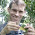## Tuesday, March 12, 2013

### Investigating whether the rate of one continuous trait is influenced by the state of another (a somewhat ad hoc approach)

Yesterday, the following query was submitted to the R-SIG-phylo email listserve:

I have a continuous dependent variable (e.g. range size) and a few "independent" variables (e.g. body mass, encephalization ratio), and I want to test how the rate of evolution of the dependent variable is affected by the independent variables. The PCMs that I'm familiar with cannot be used to answer this question, because they usually try to predict the dependent variable based on the independent variables (e.g. PGLM) instead of looking at the rates of evolution.

Ideally, we'd take a model-based approach to this problem, as pointed out by Matt Pennell. Unfortunately, this has not yet been done. In its stead, however, it is not too difficult to devise a somewhat ad hoc, simulation-based alternative. Here was my proposal:

What about the admittedly ad hoc approach of computing the correlation between the states at ancestral nodes for x & the squared contrasts for corresponding nodes for y? Then you can generate a null distribution for the test statistic (say, a Pearson or Spearman rank correlation) by simulation. This seems to give reasonable type I error when the null is correct, and when I simulate under the alternative (i.e., the rate of Brownian evolution along a branch depends on the state at the originating node) it sometimes is significant.

Here is the function I proposed and submitted to the list to do this, I have since posted a more sophisticated method & function here.

ratebystate<-function(tree,x,y,nsim=100,method=c("pearson","spearman")){
method<-method
if(!is.binary.tree(tree)) tree<-multi2di(tree)
V<-phyl.vcv(cbind(x,y),vcv(tree),lambda=1)\$R
a<-fastAnc(tree,x)
b<-pic(y,tree)[names(a)]^2
r<-cor(a,b,method=method)
beta<-setNames(lm(b~a)\$coefficients,NULL)
foo<-function(tree,V){
XY<-sim.corrs(tree,V)
a<-fastAnc(tree,XY[,1])
b<-pic(XY[,2],tree)[names(a)]^2
r<-cor(a,b,method=method)
return(r)
}
r.null<-c(r,replicate(nsim-1,foo(tree,V)))
P<-mean(abs(r.null)>=abs(r))
return(list(beta=beta,r=r,P=P,method=method))
}

I was naturally somewhat curious about how it would do. To figure that out, we need to simulate under the null & alternative models. The null model is trivial, of course - constant-rate Brownian motion. The alternative model is a little trickier. Here, I see two different alternatives: (1) the rate of branches descended from each internal node is a linear function of the state at that node; or (2) the rate of each branch is a linear function of the average state along that edge, where we compute the average as the mean of the rootward and tipwards nodes subtending the edge. [**Note that in the simple function above, we explicitly assume (1); however in the full version I allow for either model to be assumed using method="by.node" for (1), and method="by.branch" for (2).]

Let's first simulate under the proposed alternative model and see if the result is reasonable. I will show option (2) here:

> require(phytools)
> # simulate tree
> tree<-pbtree(n=200,scale=1)
> # simulate continuous independent variable
> x<-fastBM(tree,internal=TRUE)
> # now *scale* the branch lengths of the tree
> # by the mean of x for each edge
> ss<-rowMeans(matrix(x[tree\$edge],nrow(tree\$edge),2))
> zz<-tree; zz\$edge.length<-zz\$edge.length*(ss-min(ss))
> # visualize the scaled tree by trait relationship
> phenogram(zz,x,type="b",color="blue",ftype="off", xlab="expected variance",ylab="independent variable (x)")
> # now simulate y
> y<-fastBM(zz)
> # now let's fit our model
> source("ratebystate.R")
> ratebystate(tree,x[tree\$tip.label],y,method="by.branch")
\$beta
 1.169129
\$r
 0.3187849
\$P
 0.01
\$corr
 "pearson"
\$method
 "by.branch"

Well, one simulation test does not a new method make. Here's some code that I used to run simulations under the null & alternative models [actually here under model (1), from above]:
# simulate under the null
f.null<-function(){
tree<-pbtree(n=100)
x<-fastBM(tree)
y<-fastBM(tree)
ratebystate(tree,x,y,message=FALSE)
}
sim.null<-t(replicate(400,f.null()))

# simulate under the alternative (model 1)
f.alt<-function(){
tree<-pbtree(n=100)
x<-fastBM(tree,internal=TRUE)
nodes<-x[1:tree\$Nnode+length(tree\$tip.label)]
zz<-tree; zz\$edge.length<-zz\$edge.length*(nodes[as.character(tree\$edge[,1])]-min(nodes))
y<-fastBM(zz)
ratebystate(tree,x[tree\$tip.label],y,message=FALSE)
}
sim.alt<-t(replicate(400,f.alt()))

And here are the results:
> colMeans(sim.null)
beta           r           P
0.006199303 0.004057295 0.516675000
> mean(sim.null[,"P"]<0.05)
 0.03
> colMeans(sim.alt)
beta         r         P
1.0114919 0.2617357 0.0742000
> mean(sim.alt[,"P"]<0.05)
 0.6775

So it would seem (based on these very limited simulations) that the method has type I error near or below the nominal level. Furthermore, the power is not too bad when the alternative hypothesis is correct. Finally - the mean slope of the regression between contrasts variance and ancestral states is actually very close to our generating relationship - in this case 1.0.

That's it.

1.Liam,

Obviously, as you say, this clearly does not represent a full exploration of parameter space, but this seems to work (perhaps somewhat surprisingly) well. Thanks for the post. (Just posted this to Google+ to spread the word). I think the other responses to the original post are also pretty clever and deserve a second look.

matt

1.Thanks Matt.

2.See a follow-up & update here.

Note: due to the very large amount of spam, all comments are now automatically submitted for moderation.